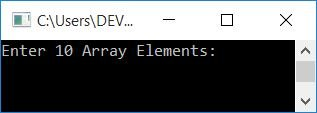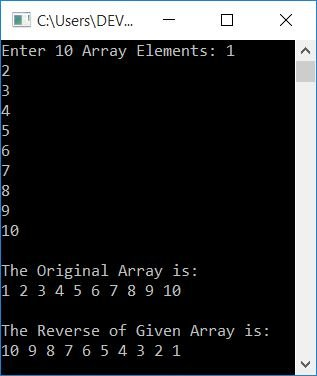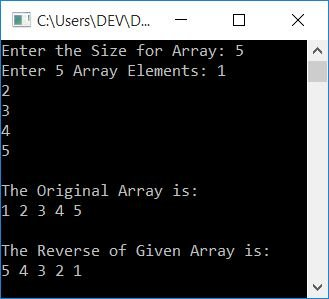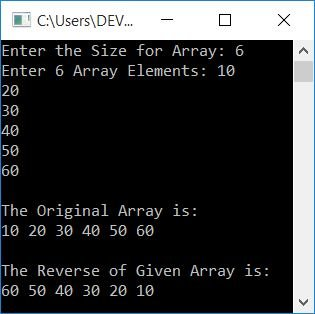# C++ Program to Reverse an Array

This article will teach you how to reverse an array entered by the user during runtime in a C++ program. Here is the list of programs for reversing an array:

## Print Reverse of an Array in C++

This program just prints reverse of an array, without actually reversing it. The question is, write a program in C++ to print reverse of an array. Here is its answer:

```#include<iostream>
using namespace std;
int main()
{
int arr, i;
cout<<"Enter 10 Array Elements: ";
for(i=0; i<10; i++)
cin>>arr[i];
cout<<"\nThe Original Array is:\n";
for(i=0; i<10; i++)
cout<<arr[i]<<" ";
cout<<"\n\nThe Reverse of Given Array is:\n";
for(i=(10-1); i>=0; i--)
cout<<arr[i]<<" ";
cout<<endl;
return 0;
}```

This program was built and runs under the Code::Blocks IDE. Here is its sample run:Now supply 10 numbers as 10 elements for an array, one by one, to print its reverse as shown in the sample output given below:When user enters any 10 numbers say 1, 2, 3, ..., 10, then these numbers gets stored in arr[] in following way:

• arr=1
• arr=2
• arr=3
• and so on up until
• arr=10

We printed the array from last to first, that is, from 9th to 0th index, to demonstrate the reverse of an array as output.

## Reverse an Array in C++

This program reverses an array before printing. When the array gets reversed, then when you print the same array, it shows its elements in reverse order. This program also allows the user to define the size of the array.

To reverse an array in C++ programming, you have to ask the user to enter the array's size and then add elements (of the given size) to the array. Now to reverse, do these things:

• Move the element at the first index to the last, and the element at the last index to the first.
• Move the element at the second index to the second last, and the element at the second last index to the second.
• and so on.

Let's have a look at the program first; its explanation is given later on:

```#include<iostream>
using namespace std;
int main()
{
int arr, tot, i, j, temp;
cout<<"Enter the Size for Array: ";
cin>>tot;
cout<<"Enter "<<tot<<" Array Elements: ";
for(i=0; i<tot; i++)
cin>>arr[i];
cout<<"\nThe Original Array is:\n";
for(i=0; i<tot; i++)
cout<<arr[i]<<" ";
j = tot-1;
for(i=0; i<j; i++, j--)
{
temp = arr[i];
arr[i] = arr[j];
arr[j] = temp;
}
cout<<"\n\nThe Reverse of Given Array is:\n";
for(i=0; i<tot; i++)
cout<<arr[i]<<" ";
cout<<endl;
return 0;
}```

Here is its sample run, with the following user input:

• 5 in size
• 1, 2, 3, 4, 5 as five array elements.

After supplying exactly these inputs, press the ENTER key to reverse the array and print both the original and its reverse, as shown in the snapshot given below:The following is the dry run of the above C++ program with the same user input (as provided in the previous output):

• tot = 5 at the start. Since I've already told about storing array elements. So arr = 1, arr = 2, arr = 3, arr = 4, and arr = 5.
• Now the original array gets printed using a for loop.
• After printing the original array, tot-1 or 5-1 or 4 gets initialized to j. So j=4
• Now 0 gets initialized to i, and the condition i<j or 0<4 evaluates to be true. Therefore, program flow goes inside the loop.
• And arr[i] or arr or 1 is set to temp. Then arr[j] or arr or 5 gets initialized to arr[i] or arr. Finally, temp or 1 is assigned to arr[j] or arr.
• A normal swap operation gets performed with three lines of code. Now arr = 5 and arr = 1. As you can see, the element at the last index (4th index) gets moved to the first index (0th index), and the element at the first (0th) index gets moved to the last (4th) index.
• Now the program flow goes to the update part of the for loop, and the value of i gets incremented, whereas the value of j gets decremented. So i = 1 and j = 3.
• And then the condition gets evaluated again. Because the condition i<j or 1<3 evaluates to be true again, program flow goes inside the loop again.
• This process continues until the condition is evaluated as false.
• In this way, the array gets reversed. So print the array using another for loop to show the reverse of the given array.

## Reverse an Array using Pointers in C++

Now let's reverse an array using a pointer. We've created a total of three programs using pointers to reverse an array given by the user. This program uses pointers everywhere, from printing to reversing an array:

```#include<iostream>
using namespace std;
int main()
{
int arr, tot, i, arrTemp, *ptr;
cout<<"Enter the Size for Array: ";
cin>>tot;
cout<<"Enter "<<tot<<" Array Elements: ";
for(i=0; i<tot; i++)
cin>>arr[i];
ptr = &arr;
cout<<"\nThe Original Array is:\n";
for(i=0; i<tot; i++)
{
cout<<*ptr<<" ";
ptr++;
}
ptr--;
for(i=0; i<tot; i++)
{
arrTemp[i] = *ptr;
ptr--;
}
ptr = &arrTemp;
for(i=0; i<tot; i++)
{
arr[i] = *ptr;
ptr++;
}
ptr = &arr;
cout<<"\n\nThe Reverse of Given Array is:\n";
for(i=0; i<tot; i++)
{
cout<<*ptr<<" ";
ptr++;
}
cout<<endl;
return 0;
}```

Here is its sample run with user input, 6 as size, and 10, 20, 30, 40, 50, and 60 as its six elements:Note: The * is called as the value at operator, whereas & is called as the address of operator.

Note: The ptr++ initializes the address of the next index. For example, if ptr holds the address of the 0th index of an array arr, then after executing ptr++, ptr now holds the address of the 1st index of the same array arr.

Here is another program using a pointer to reverse an array. In this program, the pointer is used only to reverse the array. The printing of the original and reversed arrays is done normally:

```#include<iostream>
using namespace std;
int main()
{
int arr, tot, i, arrTemp, *ptr;
cout<<"Enter the Size for Array: ";
cin>>tot;
cout<<"Enter "<<tot<<" Array Elements: ";
for(i=0; i<tot; i++)
cin>>arr[i];
cout<<"\nThe Original Array is:\n";
for(i=0; i<tot; i++)
cout<<arr[i]<<" ";
ptr = &arr[tot-1];
for(i=0; i<tot; i++)
{
arrTemp[i] = *ptr;
ptr--;
}
ptr = &arrTemp;
for(i=0; i<tot; i++)
{
arr[i] = *ptr;
ptr++;
}
cout<<"\n\nThe Reverse of Given Array is:\n";
for(i=0; i<tot; i++)
cout<<arr[i]<<" ";
cout<<endl;
return 0;
}```

This program produces the same output as the previous program. Now here is the last program that reverses an array using a pointer. This program is based on swapping operations. This program doesn't use any other array like the previous program:

```#include<iostream>
using namespace std;
int main()
{
int arr, tot, i, j, temp, *ptrOne, *ptrTwo;
cout<<"Enter the Size for Array: ";
cin>>tot;
cout<<"Enter "<<tot<<" Array Elements: ";
for(i=0; i<tot; i++)
cin>>arr[i];
cout<<"\nThe Original Array is:\n";
for(i=0; i<tot; i++)
cout<<arr[i]<<" ";
ptrOne = &arr;
ptrTwo = &arr[tot-1];
j = tot-1;
for(i=0; i<j; i++, j--)
{
temp = *ptrOne;
*ptrOne = *ptrTwo;
*ptrTwo = temp;
ptrOne++;
ptrTwo--;
}
cout<<"\n\nThe Reverse of Given Array is:\n";
for(i=0; i<tot; i++)
cout<<arr[i]<<" ";
cout<<endl;
return 0;
}```

## Reverse an Array using a Function in C++

This is the end of this article. This program is created using a user-defined function, revArray(), to reverse an array entered by the user. The function takes two arguments. The first argument is an array, and the second is its size.

```#include<iostream>
using namespace std;
void revArray(int [], int);
int main()
{
int arr, tot, i, j, temp;
cout<<"Enter the Size for Array: ";
cin>>tot;
cout<<"Enter "<<tot<<" Array Elements: ";
for(i=0; i<tot; i++)
cin>>arr[i];
cout<<"\nThe Original Array is:\n";
for(i=0; i<tot; i++)
cout<<arr[i]<<" ";
revArray(arr, tot);
cout<<"\n\nThe Reverse of Given Array is:\n";
for(i=0; i<tot; i++)
cout<<arr[i]<<" ";
cout<<endl;
return 0;
}
void revArray(int a[], int t)
{
int i, j, temp;
j = t-1;
for(i=0; i<j; i++, j--)
{
temp = a[i];
a[i] = a[j];
a[j] = temp;
}
}```

This program does exactly the same job as the previous program.

#### The same program in different languages

C++ Quiz

« Previous Program Next Program »